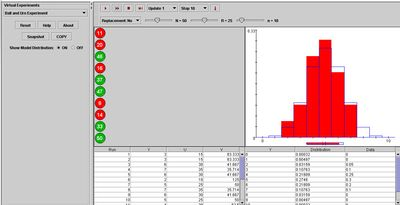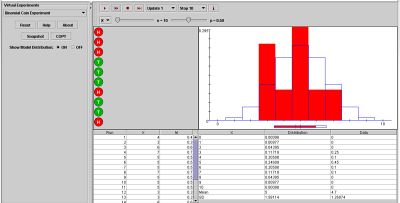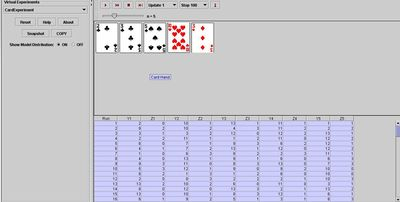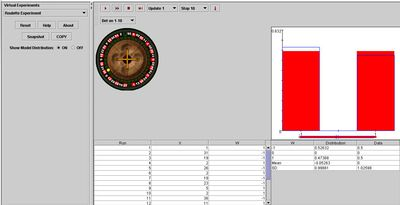# Difference between revisions of "AP Statistics Curriculum 2007 Prob Simul"

## General Advance-Placement (AP) Statistics Curriculum - Probability Theory Through Simulation

### Motivation

Many practical examples require probability computations of complex events. Such calculations may be carried out exactly, using the proper probability rules, or approximately using estimation or simulations.

A very simple example is the case of trying to estimate the area of a region, A, embedded in a square of size 1. The area of the region depends on the demarcation of its boundary, as a simple closed curve, as shown on the picture. This problem relates to the problem of computing the probability of the event A as a subset of the sample-space S - square of size 1. In other words, if we were to throw a dart at the square, S, what would be the chance that the dart land inside A (under certain conditions, e.g., the dart must land in S and each location of S is equally likely to be hit by the dart)?

This problem may be solved exactly by using integration, but an easier approximate solution would be throwing 100 darts at the board and recording the proportion of darts that landed inside A. This proportion will be a good simulation-based approximation to the real size (or probability) of the set (or event) A. For the instance of throwing 15 darts and having 7 land inside of A, the simulation-based estimate of the area (or probability) of A is $$P(A) \approx {7 \over 15}$$.

### SOCR simulations

There are a large number of SOCR Simulations that may be used to compute (approximately) probabilities of various processes and compare these empirical probabilities to their exact counterparts.

#### Ball and Urn Experiment

This experiment allows sampling with or without replacement sampling from a virtual urn that has one of two types of balls - red (successes) and green (failures). The user may control the total number of balls in the urn (N), the proportion of red to green balls (R) and the number of balls sampled from the urn (n). Depending on whether we sample with or without replacement, the chance (or probability) of getting n red balls (successes) in a sample of n balls ($$1 \geq n \geq N$$) changes. The applet records numerically the empirical outcomes and compares these to the theoretical expected counts using distribution tables and graphs. Suppose we set N=50, R=25 and n=10. How many successes (red ball) do we expect to get in the sample of 10 balls, if we sample without replacement? Hypergeometric distribution provides the theoretical model for experiment and allows us to compute this probability exactly. We can also run the experiment once and approximate this answer by dividing the number of observed red balls by 10 (the sample size). You can try this experiment gauge the accuracy of the simulation-based approximations relative to various setting, e.g., sample size. According to the Law of Large Numbers, the accuracy of the estimation will rapidly increase with the increase of the sample-size (n).#### Binomial Coin Toss Experiment

This experiment allows tossing of n independent coins, each with the probability of heads p. This may be a perfect model for any experiment that involves observing independent dichotomous measurement (e.g., success/failure, +/-, pro/con, up/down, presence/absence, etc.) where all measurements have the same fixed chance of success. Just like with the Ball and Urn experiment above, we can carry a simulation and estimate the probability of the coin being tosses by running n trials and computing the quotient of (number of successes)/n. Imagine that some one provided you only with the n outcomes (a sequence of Head and Tails), you will be able to estimate the P(H) of the process that generated these data. Using the graph and numerical table included in this applet, we can also compare the theoretical binomial probabilities against their empirical estimates.#### Card Experiment

This experiment is more involved because the sample space is significantly larger. It demonstrates the basic properties of dealing n cards at random from a standard deck of 52 cards. At every trial, n cards are randomly drawn and their denomination and suit are recorded in the result table below. We can use this simulation to estimate the probabilities of various hands (e.g., the odds of getting a pair of cards with the same denomination). Run the experiment 100 times and count the number of 5-card hands that had at least one pair in them (at least one pair of cars in the 5-card had had a matching denomination, i.e., $$Y_i=Y_j$$, for $$1 \leq i < j \leq 5$$). Dividing this number by 100 gives the simulation-based estimate of the probability of the complex event of interest (at least one pair).#### Roulette Experiment

The Roulette experiment presents another interesting example where we can draw direct synergies between exact theoretical probability and approximate simulation probability calculations. The Roulette wheel has 38 slots numbered 00, 0, and 1-36. Slots 00 and 0 are green. Half of the slots numbered 1-36 are red and half are black. Suppose we are interested in the odds of winning (i.e., the probability of the event) if we bet on a number between 1-18 turns up. Obviously, the chance of winning is given by the fraction 18/38 (which is less than 50%). However, we can run this experiment 20 times and the empirical number of time we win could be different each time, yet close to the theoretical value of 18/38. In the image below we won 10 out of the 20 trials and therefore, the empirical odds are 50-50.